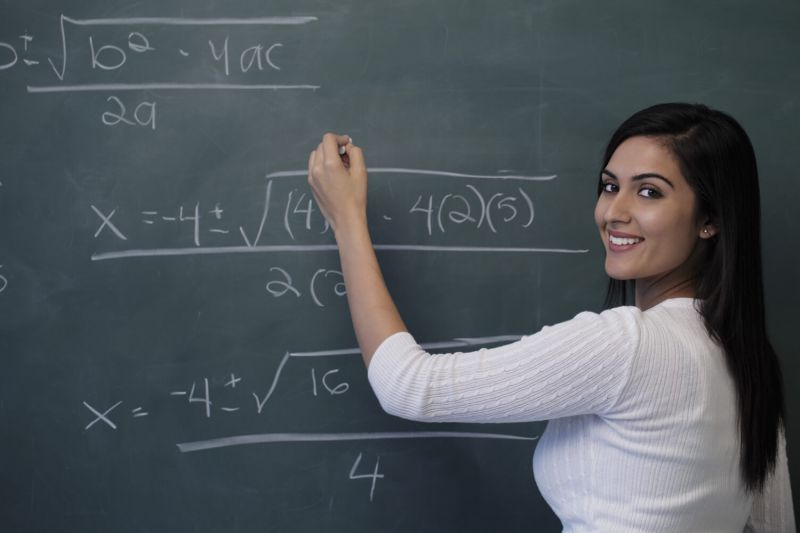Vedic Math Teacher Training & Concept-

What Is Vedic Math?
Veda is a Sanskrit word which means knowledge is a collection of sutras to solve mathematical arithmetic in easy and faster way.It consists of 16 sutras (Formulae) and 13 sub –sutras (Sub-formulae) which can be used for problems involved arithmetic,algebra,geometry,calculus& conics.
Vedic mathematics can definitely solve mathematical  numerical calculations in faster way.we can do calculations 10 times faster than conventional or our usual methods

Benefits

• Increases mental agility and intelligence
• Increases speed and accuracy
• Improves memory
• Sharpens mind
• save time
• useful for the competitive exams

In today’s competitive scenario very difficult to crack competitive exam,IItexam,CATExam,upsc exam ,etc .Vedic Mathematics reduce time in solving mathematical calculations with accuracy and speed.

Great business  Opportunity with minimum investment  for Teachers,educatedfemales,self –employed,etc  by training to senior students.

Teacher Training Duration-

• Basic-I-5-7 Days
• Basic-II-4-6 days